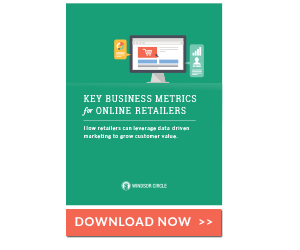# Customer Retention. AutomatedRetailers who are using the Windsor Circle Retention Marketing Platform will find numerous reports and charts that show both Average Order Value and Median Order Value.  Which leads to a great and basic question.  What's the difference?

This is a standard question in basic statistics, and you'll pick it up right away in this quick synopsis.

## Central Tendency

Both "average" and "median" refer to measures of "central tendency."

Huh?

Central tendency just means that the data tends to gather around a point.

If you see {2, 4, 6, 6, 7, 9, 14} you can visually see that the data gathers around 6 or 7.

The average of this set is 6.85.  Simply add the numbers up (48) and divide by 7 (the number of data points).  48/7 = 6.85.

The median is different.  The median is the middle of the data set (in this case, 6).  Half of the observations (7,9,14) are above 6 and half (2,4,6) are below 6.

## Applying These Concepts to Order Value

Why is this important?  It's all about the accuracy of your predictive analytics.

The reason is that we often see retailers mistakenly refer to their Average Order Value (AOV) as what is statistically a likely "middle" number...  that the typical future order will be near this number.  The reason we include Median Order Value is that there are instances where it's actually a better predictor of future order values than the average....  because the "average" can be more easily skewed by an outlier.

Take that same data set above, but change the last value to 140.  The average is now nearly 25.  The median is still 6.

And, if you were trying to predict what future observations would look like, they'd darn sure look a lot more like 6 then 25 based on this set of data.  This is because the 140 value is an outlier, unlikely to be repeated.  Assume for a second that this data set was a set of order values.  If you were asked to predict what the next 100 numbers would be if you added them up, would you say 100*6 = 600?  Or would you say 100*25 =2500?

Now imagine that those were order values, and your boss was asking, based on your predictive analytics, how much revenue you think you're going to make?  There's more than a 4x difference here depending on if you use the Average Order Value (AOV) or the Median Order Value (MOV) against the 100 predictive orders to make the calculation.

That spread is why it matters...

So, when thinking about Average Order Value (AOV), be excited about your new found ability with Windsor Circle to do a quick check and make sure that your Median Order Value (MOV) is in the same range.  If not, you'll want to at least make an informed decision about which "Measure of Central Tendency" you choose to use.

Just be sure to say "Measure of Central Tendency" in your meeting so that your boss will be really impressed!Topics: Customer Retention